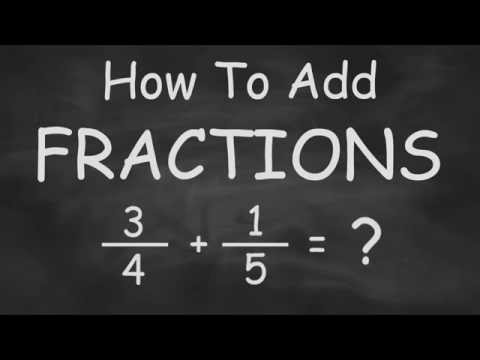Relevance
• You have to make them all into the same sort of fraction (ie have a common denominator;

eg 1/2 + 2/3 + 3/4

What number is divisible by 2, 3 and 4?

Convert them all into 12ths by dividing the bottom into 12 and multiplying it by the top:

1/2 = 6/12

2/3 = 8/12

3/4 = 9/12

Now you can add the tops together, leaving the bottom as 12ths

6/12 + 8/12 + 8/12 = 22/12

You can simplify 22/12 into 11/6

• The underlying concept is that you can add apples to apples but not apples and oranges. So you need to change the apples to oranges. In order to add fractions you need to have the same denominators (bottoms) and whatever you do to the bottoms you must do exactly the same to the numerators (tops) Here are a couple of methods:

1) Guaranteed method for Common Denominator (CD) for ALL fractions

3/4 + 1/5

Multiply the denominators together

3/4 X 5/5 + 1/5 X 4/4

15/20 + 4/20 = 19/20

2) Find the Least Common Multiple (LCM) for the denominators

Find the prime factors for the denominators. Guaranteed method for all denominators

4 = 2 X 2 X 1

5 = 5 X 1

common factor is 1

LCM

2 X 2 X 5 X 1 = 20

Common denominator is 20

3/4 X 5/5 = 15/20

1/5 X 4/4 = 4/20

3) Inspection

This method depends on how well you know and understand the multiplication tables

The first number (multiple) that is common to both 4 and 5 is 20.

Thus 20 is the common denominator

Do keep in mind that quite often one given denominator is a multiple of the other

Example: 1/3 + 1/6

Since 6 is the LCM the common denominator is 6

1/3 X 2/2 + 1/6

2/6 + 1/6 = 3/6 = 1/2

The above methods can used for the subtraction of fractions as well.